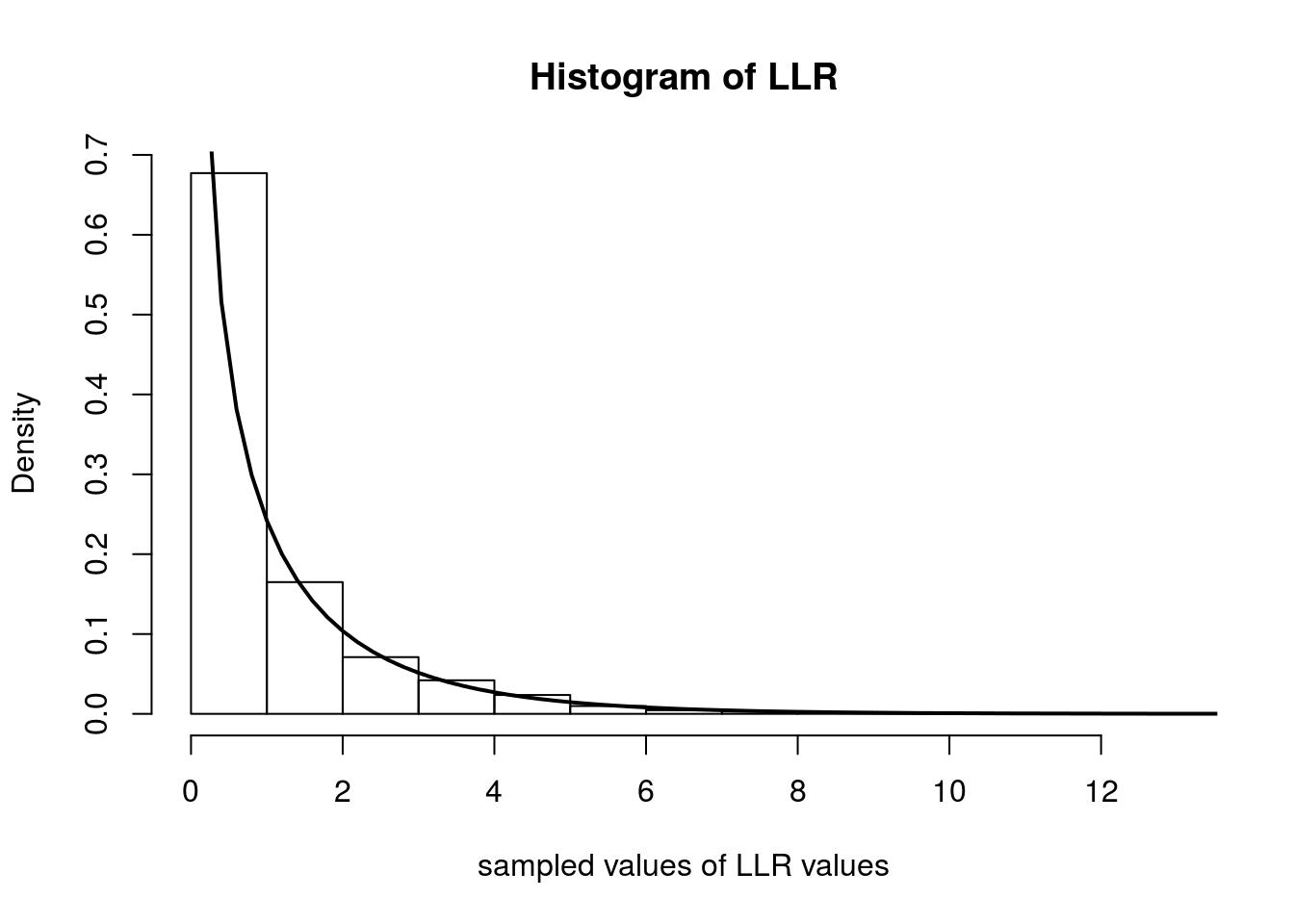Last updated: 2017-03-04

Code version: 5d0fa13282db4a97dc7d62e2d704e88a5afdb824

## Pre-requisites

This document assumes familiarity with the concepts of likelihoods, likelihood ratios, and hypothesis testing.

## Background

When performing a statistical hypothesis test, like comparing two models, if the hypotheses completely specify the probability distributions, these hypotheses are called simple hypotheses. For example, suppose we observe $$X_1,\ldots,X_n$$ from a normal distribution with known variance and we want to test whether the true mean is equal to $$\mu_0$$ or $$\mu_1$$. One hypothesis $$H_0$$ might be that the distribution has mean $$\mu_0$$, and $$H_1$$ might be that the mean is $$\mu_1$$. Since these hypotheses completely specify the distribution of the $$X_i$$, they are called simple hypotheses.

Now suppose $$H_0$$ is again that the true mean, $$\mu$$, is equal to $$\mu_0$$, but $$H_1$$ was that $$\mu > \mu_0$$. In this case, the $$H_0$$ is still simple, but $$H_1$$ does not completely specify a single probability distribution. It specifies a set of distributions, and is therefore an example of a composite hypothesis. In most practical scenarios, both hypotheses are rarely simple.

As seen in the fiveMinuteStats on likelihood ratios, given the observed data $$X_1\ldots,X_n$$, we can measure the relative plausibility of $$H_1$$ to $$H_0$$ by the log-likelihood ratio: $\log\left(\frac{f(X_1,\ldots,X_n|H_1)}{f(X_1,\ldots,X_n|H_0)}\right)$

The log-likelihood ratio could help us choose which model ($$H_0$$ or $$H_1$$) is a more likely explanation for the data. One common question is this: what constitutes are large likelihood ratio? Wilks’s Theorem helps us answer this question – but first, we will define the notion of a generalized log-likelihood ratio.

## Generalized Log-Likelihood Ratios

Let’s assume we are dealing with distributions parameterized by $$\theta$$. To generalize the case of simple hypotheses, let’s assume that $$H_0$$ specifies that $$\theta$$ lives in some set $$\Theta_0$$ and $$H_1$$ specifies that $$\theta \in \Theta_1$$. Let $$\Omega = \Theta_0 \cup \Theta_1$$. A somewhat natural extension to the likelihood ratio test statistic we discussed above is the generalized log-likelihood ratio: $\Lambda^* = \log{\frac{\max_{\theta \in \Theta_1}f(X_1,\ldots,X_n|\theta)}{\max_{\theta \in \Theta_0}f(X_1,\ldots,X_n|\theta)}}$

For technical reasons, it is preferable to use the following related quantity:

$\Lambda_n = 2\log{\frac{\max_{\theta \in \Omega}f(X_1,\ldots,X_n|\theta)}{\max_{\theta \in \Theta_0}f(X_1,\ldots,X_n|\theta)}}$

Just like before, larger values of $$\Lambda_n$$ provide stronger evidence against $$H_0$$.

## Wilks’s Theorem

Suppose that the dimension of $$\Omega = v$$ and the dimension of $$\Theta_0 = r$$. Under regularity conditions and assuming $$H_0$$ is true, the distribution of $$\Lambda_n$$ tends to a chi-squared distribution with degrees of freedom equal to $$v-r$$ as the sample size tends to infinity.

With this theorem in hand (and for $$n$$ large), we can compare the value of our log-likehood ratio to the expected values from a $$\chi^2_{v-r}$$ distribution.

## Example: Poisson Distribution

Assume we observe data $$X_1,\ldots X_n$$ and consider the hypotheses $$H_0: \lambda = \lambda_0$$ and $$H_1: \lambda \neq \lambda_0$$. The likelihood is: $L(\lambda|X_1,\ldots,X_n) = \frac{\lambda^{\sum X_i}e^{-n\lambda}}{\prod_i^n X_i!}$

Note that $$\Theta_1$$ in this case is the set of all $$\lambda \neq \lambda_0$$. In the numerator of the expression for $$\Lambda_n$$, we seek $$\max_{\theta \in \Omega}f(X_1,\ldots,X_n|\theta)$$. This is just the maximum likelihood estimate of $$\lambda$$ which we derived in this note. The MLE is simply the sample average $$\bar{X}$$. The likelihood ratio is therefore: $\frac{L(\lambda=\bar{X}|X_1,\ldots,X_n)}{L(\lambda=\lambda_0|X_1,\ldots,X_n)} = \frac{\bar{X}^{\sum X_i}e^{-n\bar{X}}}{\prod_i^n X_i!}\frac{\prod_i^n X_i!}{\lambda_0^{\sum X_i}e^{-n\lambda_0}} = \big ( \frac{\bar{X}}{\lambda_0}\big )^{\sum_i X_i}e^{n(\lambda_0 - \bar{X})}$

which means that $$\Lambda_n$$ is $\Lambda_n = 2\log{\left( \big ( \frac{\bar{X}}{\lambda_0}\big )^{\sum_i X_i}e^{n(\lambda_0 - \bar{X})} \right )} = 2n \left ( \bar{X}\log{\left(\frac{\bar{X}}{\lambda_0}\right)} + \lambda_0 - \bar{X} \right )$

In this example we have that $$v$$, the dimension of $$\Omega$$, is 1 (any positive real number) and $$r$$, the dimension of $$\Theta_0$$ is 0 (it’s just a single point). Hence, the degrees of freedom of the asymptotic $$\chi^2$$ distribution is $$v-r = 1$$. Therefore, Wilk’s Theorem tells us that $$\Lambda_n$$ tends to a $$\chi^2_1$$ distribution as $$n$$ tends to infinity.

Below we simulate computing $$\Lambda_n$$ over 5000 experiments. In each experiment, we observe 500 random variables distributed as Poisson($$0.4$$). We then plot the histogram of the $$\Lambda_n$$s and overlay the $$\chi^2_1$$ density with a solid line.

num.iterations         <- 5000
lambda.truth           <- 0.4
num.samples.per.iter   <- 500
samples                <- numeric(num.iterations)
for(iter in seq_len(num.iterations)) {
data            <- rpois(num.samples.per.iter, lambda.truth)
samples[iter]   <- 2*num.samples.per.iter*(mean(data)*log(mean(data)/lambda.truth) + lambda.truth - mean(data))
}
hist(samples, freq=F, main='Histogram of LLR', xlab='sampled values of LLR values')
curve(dchisq(x, 1), 0, 20, lwd=2, xlab = "", ylab = "", add = T)sessionInfo()
R version 3.3.0 (2016-05-03)
Platform: x86_64-apple-darwin13.4.0 (64-bit)
Running under: OS X 10.10.5 (Yosemite)

locale:
 en_NZ.UTF-8/en_NZ.UTF-8/en_NZ.UTF-8/C/en_NZ.UTF-8/en_NZ.UTF-8

attached base packages:
 stats     graphics  grDevices utils     datasets  methods   base

other attached packages:
 tidyr_0.4.1     dplyr_0.5.0     ggplot2_2.1.0   knitr_1.15.1
 MASS_7.3-45     expm_0.999-0    Matrix_1.2-6    viridis_0.3.4
 workflowr_0.3.0 rmarkdown_1.3

loaded via a namespace (and not attached):
 Rcpp_0.12.5      git2r_0.18.0     plyr_1.8.4       tools_3.3.0
 digest_0.6.9     evaluate_0.10    tibble_1.1       gtable_0.2.0
 lattice_0.20-33  shiny_0.13.2     DBI_0.4-1        yaml_2.1.14
 gridExtra_2.2.1  stringr_1.2.0    gtools_3.5.0     rprojroot_1.2
 grid_3.3.0       R6_2.1.2         reshape2_1.4.1   magrittr_1.5
 backports_1.0.5  scales_0.4.0     htmltools_0.3.5  assertthat_0.1
 mime_0.5         colorspace_1.2-6 xtable_1.8-2     httpuv_1.3.3
 labeling_0.3     stringi_1.1.2    munsell_0.4.3   

This site was created with R Markdown